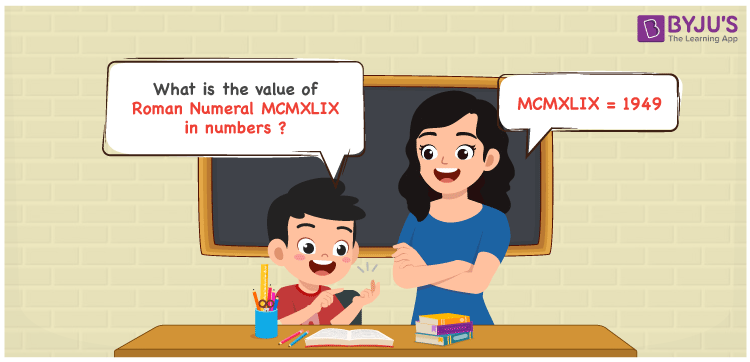Checkout JEE MAINS 2022 Question Paper Analysis : Checkout JEE MAINS 2022 Question Paper Analysis :

# MCMXLIX Roman Numerals

MCMXLIX Roman Numerals is 1949. Roman Numeral is the technique of writing numbers using the Roman alphabet. Practising the problems referring to the PDF of Roman Numerals 1 to 100 helps students to enhance time management and problem-solving skills which are crucial from an exam point of view. In this article, let us learn how to denote the number form of MCMXLIX Roman Numerals using simple tricks.

 Number Roman Numeral 1949 MCMXLIX

## How to Write MCMXLIX Roman Numerals?Following methods can be used to represent the numerical value of MCMXLIX Roman Numerals

First Method:

• Break the Roman Numerals into single letters
• MCMXLIX = M + (M – C) + (L – X) + (X – I)
• Write the numerical value of each letter and add/subtract them
• MCMXLIX = 1000 + (1000 – 100) + (50 – 10) + (10 – 1) = 1949

Second Method:

In this method, we consider the groups of Roman Numerals for addition or subtraction

• MCMXLIX = M + CM + XL + IX = 1000 + 900 + 40 + 9 = 1949
• Thus the numerical value of MCMXLIX Roman Numerals is 1949

## Video Lesson on Roman Numerals## Related Articles

Roman Numerals

Roman Numerals 1 to 50

Roman Numerals 1 to 1000

Roman Numerals Conversion

L Roman Numerals

Roman Numeral XXXIX

## Frequently Asked Questions on MCMXLIX Roman Numerals

### Write the Roman Numeral MCMXLIX in numbers.

Roman Numeral MCMXLIX in numbers is written as 1949.

### Express the sum of 1900 and 49 in Roman Numerals.

1900 + 49 = 1949. The number 1949 in Roman Numerals is written as MCMXLIX. Therefore the sum of 1900 and 49 in Roman Numerals is MCMXLIX.

### What is the difference between 1949 and 930 in Roman Numerals?

1949 – 930 = 1019. The number 1019 in Roman Numerals is MXIX. Hence the difference between 1949 and 930 in Roman Numerals is MXIX.